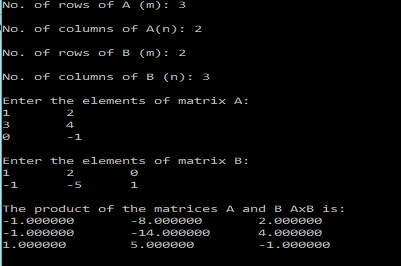# C Program to multiply two matrices

One can define matrices in C using 2-D arrays.

In this post I will assume, that you are familiar with the concepts of arrays.

In the last post I showed you guys how to sum/subtract to matrices of the same order.

In this post I will show you how to write a C program that gives the product of two matrices.

The product of two matrices isn’t always defined.
The product of matrices$A$ and$B$:$A\times B$ is defined only when the no. of columns of$A$ is equal to the no. of rows in matrix$B$.

If$A$ is an$m\times p$ matrix, and$B$ is an$p\times n$ matrix, then the product matrix would be a$m\times n$ matrix,$(A\times B)_{ij} = \sum_{k=1}^pA_{ik}B_{kj}$

With the above, information, we can proceed to write a simple program, to multiply two matrices of given sizes.
We would also need to check whether the matrix product is defined or not.

The program is pretty much self-explanatory.

### PROGRAM:

/**************************************************
*************MATRIX MULTIPLICATION*****************
**************************************************/
#include<stdio.h>
int main(){
int m1,n1,m2,n2,m,n,i,j,k;
printf("Enter the size of the matrices:\nNo. of rows of A (m): ");
scanf("%d",&m1);
printf("\nNo. of columns of A(n): ");
scanf("%d",&n1);
printf("\nNo. of rows of B (m): ");
scanf("%d",&m2);
printf("\nNo. of columns of B (n): ");
scanf("%d",&n2);
if(n1!=m2){
printf("\nThe matrices can't be multiplied.'");
//Exit the program
return 0;
}
m=m1;
n=n2;
double a[m][n1];
double b[n1][n];
double prod[m][n];
printf("\nEnter the elements of matrix A:\n");
for(i=0;i<m;i++){
for(j=0;j<n1;j++){
scanf("%lf",&a[i][j]);
}
}
printf("\nEnter the elements of matrix B:\n");
for(i=0;i<n1;i++){
for(j=0;j<n;j++){
scanf("%lf",&b[i][j]);
}
}
for(i=0;i<m;i++){
for(j=0;j<n;j++){
prod[i][j]=0;
for(k=0;k<n1;k++){
prod[i][j]=prod[i][j]+a[i][k]*b[k][j];
}
}
}
printf("\nThe product of the matrices A and B AxB is:\n");
for(i=0;i<m;i++){
for(j=0;j<n;j++){
printf("%lf \t",prod[i][j]);
}
printf("\n");
}
}

### OUTPUT:

The program output is shown below for some sample matrices:The above program, could be made cleaner and better by using separate functions for reading and printing the matrices, as well as calculating the product.

For the function that calculates the product, I would have preferred to have the function return the matrix product, but unfortunately, C doesn’t support returning arrays from functions.

So we are left with two choices:
1. We create a matrix called prod in our main program and then pass it as a parameter to the product function, which would then populate this matrix with the product. Note, that in C when you pass an array as a parameter, you are not passing it by value like it happens with variables, but rather you are passing a reference to the array itself. So when you pass the matrix that stores the product, the original matrix will be modified. All of this can work out for us without much hassle.
2. Another, option would be to use pointers. We would use malloc to create our product matrix in the function to allocate sufficient space. And then return the pointer to this array. This would be a dynamic allocation.
In this post I will be going with the first method, due to it’s simplicity, and no use of pointers.

The following codes illustrate the above procedure to multiply two matrices.

### CODE:

/**************************************************
*************MATRIX MULTIPLICATION*****************
**************************************************/
#include<stdio.h>
/*******
Function that calculates the product of two matrices:
There are two options to do this in C.
1. Pass a matrix (prod) as the parameter, and calculate and store the product in it.
2. Use malloc and make the function of pointer type and return the pointer.
This program uses the first option.
********/
void matProduct(int m, int n, int n1,double a[m][n1], double b[n1][n], double prod[m][n]){
int i,j,k;
for(i=0;i<m;i++){
for(j=0;j<n;j++){
prod[i][j]=0;
for(k=0;k<n1;k++){
prod[i][j]=prod[i][j]+a[i][k]*b[k][j];
}
}
}
}
/*******
Function that reads the elements of a matrix row-wise
Parameters: rows(m),columns(n),matrix[m][n]
*******/
void readMatrix(int m, int n, double matrix[m][n]){
int i,j;
for(i=0;i<m;i++){
for(j=0;j<n;j++){
scanf("%lf",&matrix[i][j]);
}
}
}
/*******
Function that prints the elements of a matrix row-wise
Parameters: rows(m),columns(n),matrix[m][n]
*******/
void printMatrix(int m, int n, double matrix[m][n]){
int i,j;
for(i=0;i<m;i++){
for(j=0;j<n;j++){
printf("%lf\t",matrix[i][j]);
}
printf("\n");
}
}
int main(){
int m1,n1,m2,n2,m,n,i,j;
printf("Enter the size of the matrices:\nNo. of rows of A (m): ");
scanf("%d",&m1);
printf("\nNo. of columns of A(n): ");
scanf("%d",&n1);
printf("\nNo. of rows of B (m): ");
scanf("%d",&m2);
printf("\nNo. of columns of B (n): ");
scanf("%d",&n2);
if(n1!=m2){
printf("\nThe matrices can't be multiplied.'");
//Exit the program
return 0;
}
m=m1;
n=n2;
double a[m][n1];
double b[n1][n];
double prod[m][n];
printf("\nEnter the elements of matrix A:\n");